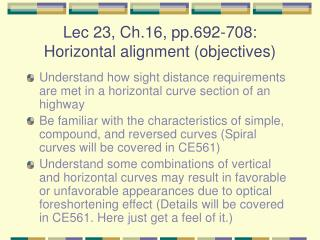DownloadDownload PresentationLec 23, Ch.16, pp.692-708: Horizontal alignment (objectives)

# Lec 23, Ch.16, pp.692-708: Horizontal alignment (objectives)

Télécharger la présentation## Lec 23, Ch.16, pp.692-708: Horizontal alignment (objectives)

- - - - - - - - - - - - - - - - - - - - - - - - - - - E N D - - - - - - - - - - - - - - - - - - - - - - - - - - -
##### Presentation Transcript

1. Lec 23, Ch.16, pp.692-708: Horizontal alignment (objectives) • Understand how sight distance requirements are met in a horizontal curve section of an highway • Be familiar with the characteristics of simple, compound, and reversed curves (Spiral curves will be covered in CE561) • Understand some combinations of vertical and horizontal curves may result in favorable or unfavorable appearances due to optical foreshortening effect (Details will be covered in CE561. Here just get a feel of it.)

2. What we cover in class today… • How to determine appropriate horizontal curve radii when sight obstructions exist • Curve equations for simple horizontal curves • Curve equations for compound horizontal curves • Curve equations for reverse horizontal curves • A few examples of the combination of horizontal and vertical curves

3. Deriving the formula for determining the middle ordinate θ θ You can determine m, R, or S using this formula, depending on the information known. This is the correct form. The one in page 707 has a typo. (Review Example 16-6)

4. Range of lower values for stopping sight distances on horizontal curves D (degree of curvature) = Central angle subtended by the 100ft arc length

5. Formulas for simple circular curves that you want to know

6. Setting out of a simple horizontal curve • Usually PI is given: • First find the station of PC  Subtract T from the station of PI • Then find the next whole station from PC. Compute l1 to find 1. • Then find D for L = 100 ft • Then find the left over of the curve length l2 to determine the station of PT T (Review Example 16-7)

7. Compound curves R1 and R2 are usually known. Eq. 16-39 and Eq. 16-40 on page 715 are missing a “bar” above VG and VH.

8. Reverse curves Reverse curves are seldom recommended. They are absolutely NOT recommended for high-speed roads. For high-speed roads, we must provide a tangent section that will allow full development of superelevation at both ends.

9. Reverse curves (2) Reverse curves usually consist of two simple curves with equal radii turning in opposite directions with a common tangent. Remember that the deflection angle is one-half of the central angle? Hence,

10. Visual effect of having a spiral curve before a simple horizontal curve The sharp “corners” at the juncture of curve and tangent section may become obvious to from the driver’s seat especially when the radius is small. Smooth transition from tangent to curve

11. Visual effect caused by the combination of horizontal and vertical curves (1)

12. Visual effect caused by the combination of horizontal and vertical curves (1a)

13. Visual effect caused by the combination of horizontal and vertical curves (2)

14. Visual effect caused by the combination of horizontal and vertical curves (3)

15. Visual effect caused by the combination of horizontal and vertical curves (4)

16. Visual effect caused by the combination of horizontal and vertical curves (5)

17. Video: “Cow Turn” A mix of low skid resistance, probably inadequate superelevation for the posted speed, and wet pavement causes… It is an entrance (on-ramp) to the Cross Bronx Expressway (I-95 in Bronx) from another expressway (off-ramp). I forgot the exact location; it is one of the circled ones